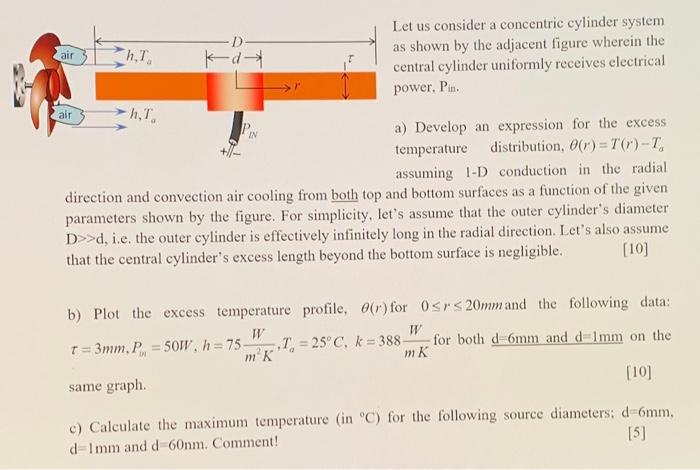# (Solved): Let us consider a concentric cylinder system as shown by the adjacent figure wherein the central cy ...Let us consider a concentric cylinder system as shown by the adjacent figure wherein the central cylinder uniformly receives electrical power, Pin. a) Develop an expression for the excess temperature distribution, assuming 1-D conduction in the radial direction and convection air cooling from both top and bottom surfaces as a function of the given parameters shown by the figure. For simplicity, let's assume that the outer cylinder's diameter d, i.e. the outer cylinder is effectively infinitely long in the radial direction. Let's also assume that the central cylinder's excess length beyond the bottom surface is negligible. b) Plot the excess temperature profile, for and the following data: for both and on the same graph. c) Calculate the maximum temperature (in ) for the following source diameters; , and . Comment! 

We have an Answer from Expert